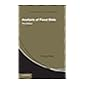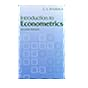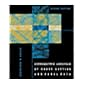Normal view MARC view ISBD view

# Numerical methods in economics / Kenneth L. Judd.

Material type:TextPublisher: Cambridge, Mass. : MIT Press, 1998Description: xiii, 633 p. : ill. ; 24 cm.ISBN: 0262100711 (hc).DDC classification: 330.015195 JUN Online resources: OCLC | Ebook Fulltext
Contents:
Part 1 Introduction: introduction; elementary concepts in numerical analysis. Part 2 Basics from numerical analysis on Rn: linear equations and iterative methods; optimization; nonlinear equations; approximation methods; numerical integration and differentiation; Monte Carlo and simulation methods; quasi-Monte Carlo methods. Part 3 Numerical methods for functional problems: finite-difference methods; projection methods for functional equations; numerical dynamic programming. Part 4 Perturbation methods: regular perturbation of simple systems; regular perturbations in multidimensional systems; advanced asymptotic methods. Part 5 Applications to dynamic equilibrium analysis; solution methods for perfect foresight models; solving rational expectations models.
Summary: To harness the full power of computer technology, economists need to use a broad range of mathematical techniques. In this book, Kenneth Judd presents techniques from the numerical analysis and applied mathematics literatures and shows how to use them in economic analyses.
Tags from this library: No tags from this library for this title.Average rating: 0.0 (0 votes)
Item type Current location Collection Call number Copy number Status Date due Barcode Item holdsE-Book
E-book
Non-fiction 330.015195 JUN 1998 (Browse shelf) Not for loanText
Reserve Section
Non-fiction 330.015195 JUN 1998 (Browse shelf) C-1 Not For Loan 25181Text
Reserve Section
Non-fiction 330.015195 JUN 1998 (Browse shelf) C-2 Not For Loan 25182Text
Circulation Section
Non-fiction 330.015195 JUN 1998 (Browse shelf) C-3 Available 25183
Total holds: 0
##### Browsing EWU Library Shelves , Shelving location: E-book Close shelf browser330.015195 GUB 2009 Basic econometrics 330.015195 HSA 2014 Analysis of panel data / 330.015195 JOE 1997 Econometric methods / 330.015195 JUN 1998 Numerical methods in economics / 330.015195 MAI 1992 Introduction to econometrics / 330.015195 STI 2011 Introduction to econometrics / 330.015195 WOE 2010 Econometric analysis of cross section and panel data /

Includes bibliographical references (p. -622) and index.

Part 1 Introduction: introduction; elementary concepts in numerical analysis. Part 2 Basics from numerical analysis on Rn: linear equations and iterative methods; optimization; nonlinear equations; approximation methods; numerical integration and differentiation; Monte Carlo and simulation methods; quasi-Monte Carlo methods. Part 3 Numerical methods for functional problems: finite-difference methods; projection methods for functional equations; numerical dynamic programming. Part 4 Perturbation methods: regular perturbation of simple systems; regular perturbations in multidimensional systems; advanced asymptotic methods. Part 5 Applications to dynamic equilibrium analysis; solution methods for perfect foresight models; solving rational expectations models.

To harness the full power of computer technology, economists need to use a broad range of mathematical techniques. In this book, Kenneth Judd presents techniques from the numerical analysis and applied mathematics literatures and shows how to use them in economic analyses.

Economics

There are no comments for this item.